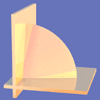Search by Topic

Resources tagged with Vector equations of plane similar to Walls:

Filter by: Content type:
Age range:
Challenge level:

There are 2 results

Broad Topics > Vectors and Matrices > Vector equations of planeWalls

Age 16 to 18 Challenge Level:

Plane 1 contains points A, B and C and plane 2 contains points A and B. Find all the points on plane 2 such that the two planes are perpendicular.Quaternions and Reflections

Age 16 to 18 Challenge Level:

See how 4 dimensional quaternions involve vectors in 3-space and how the quaternion function F(v) = nvn gives a simple algebraic method of working with reflections in planes in 3-space.